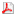Metallic Materials Vol. 48 (2010), no. 1, pp. 41 - 46

Title: High temperature plastic deformation of CuZn30 brass - calculation of the activation energy
Author: PERNIS, R., KASALA, J., BORUTA, J.

Abstract: High temperature plastic deformation of CuZn30 brass was investigated in the temperature range between 650 °C and 850 °C. Torsion tests were performed for experimental plan according to test array 5 x 4, i.e. five temperatures 650, 700, 750, 800, 850 °C and four speeds of torsion shear strain rate 0.5, 2.5, 12.5, and 25 s-1. Mathematical model has been developed to predict high-temperature shear stress behaviour of CuZn30 brass. Explicit equation, which is a function of peak stress, temperature and shear strain rate, was used in the mathematical model. There are four constants in the equation; one of those is the activation energy which for CuZn30 brass reaches the value Q=180.3 kJ mol-1. Measured and calculated values of shear stress are shown in graphs in dependence on the value of deformation, where the temperature of deformation and the speed of deformation are the parameters for the particular curves. The predicted results are in accordance with the experimental curves stress - deformation that can be used to model the behaviour of hot extrusion pressing of CuZn30 brass.

Keywords: brass CuZn30, torsion test, strain, flow stress, activation energy
Year: 2010, Volume: 48, Issue: 1 Page From: 41, Page To: 46
doi:10.4149/km_2010_1_41download file